## Pages

Showing posts with label solutions. Show all posts
Showing posts with label solutions. Show all posts

### Absolute Value Equations

Absolute value, by definition, is the distance from zero on a number line. If we have |x| = 3 then the question is, “what number can x be so that the distance to zero is 3?” There are two solutions to this question -3 and 3.
In general,
Here n is a positive integer and X represents an algebraic expression called the argument of the absolute value. To solve these equations, set the argument equal to plus or minus n and solve the resulting equations.

Instructional Video: Absolute Value Equations

Solve.
This technique requires us to first isolate the absolute value. Apply the usual steps for solving to obtain the absolute value alone on one side of the equation, and then set the argument to plus or minus n.

### Solving Equations Quadratic in Form

In this section, we make use of all the techniques that we have learned so far for solving quadratic equations. In fact, the equations found here are reducible to quadratic form.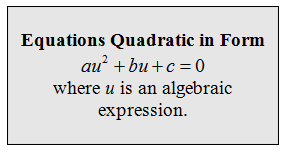Here are most of the reducible equations that we are likely to encounter. Begin by trying to identify what can be squared to obtain the leading variable term.Tip: Look at the middle term for a hint as to what u should be.In the previous solved problem, we certainly could have distributed the expression on the left side, put the equation in standard form then re-factored it. Instead, here we are illustrating a technique that will be used to easily solve many other equations that are quadratic in form.

Solve by making a u-substitution.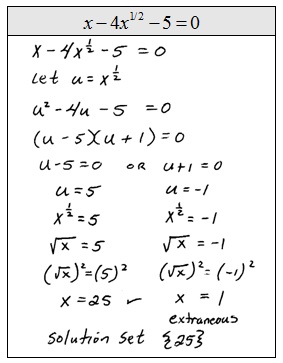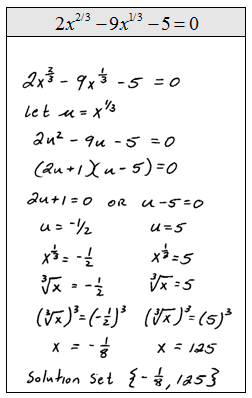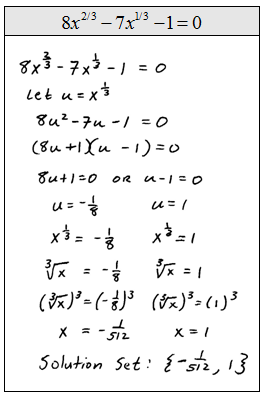Solve: x^6 + 26x^3 -27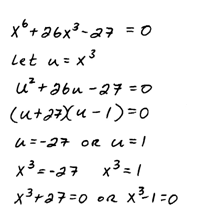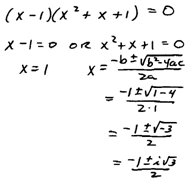Six Answers: { -3, 1, (3±3iSqrt(3))/2, (-1±iSqrt(3))/2 }

So far we have been able to factor after we make the u-substitution.  If the resulting quadratic equation does not factor, then use the quadratic formula.